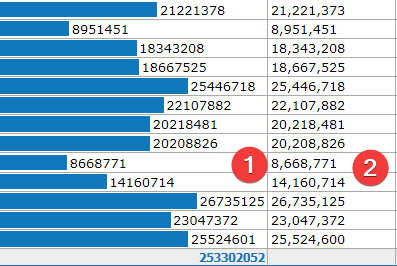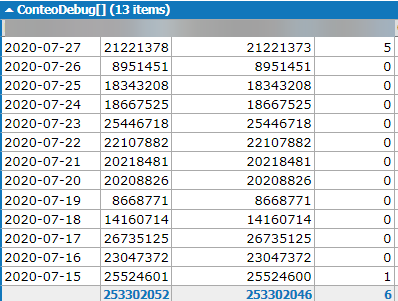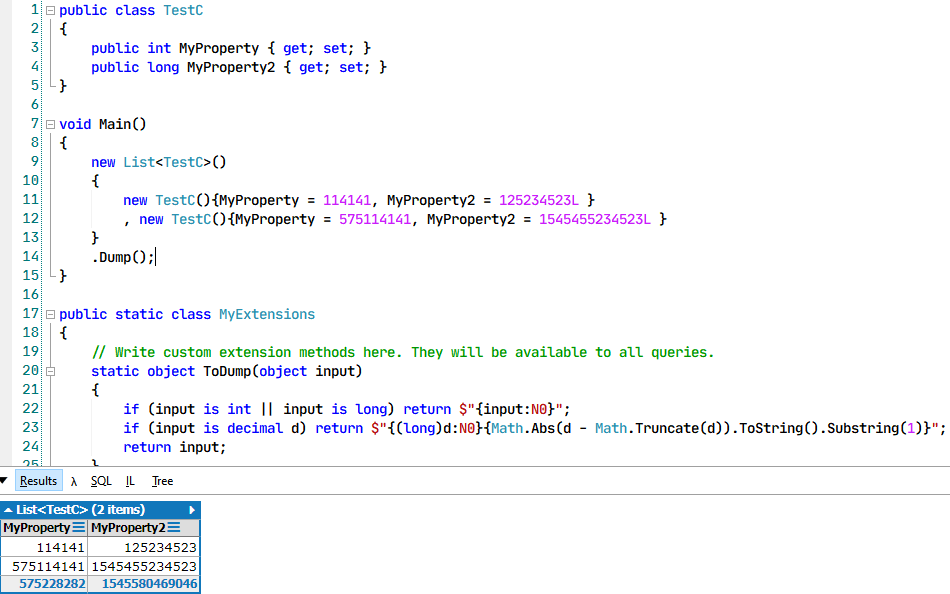# Format numbers in Dump and still get sum

Hello everyone!

I am wondering if it is possible to format numbers in the dump output (as shown in column 2) while keeping the ability to get the sum of all the values and the quantity bars as shown in column 1.

What I did to format the numbers was to cast the long value as string and use the "N0" format.Regards

• If you're happy to make this formatting the default, you can avoid the problem by doing the transformation in the ToDump method. Add the following code to My Extensions

```static object ToDump (object input)
{
if (input is int || input is long) return \$"{input:N0}";
if (input is decimal d) return \$"{(long)d:N0}{Math.Abs (d - Math.Truncate (d)).ToString().Substring(1)}";
return input;
}
```
• Thanks for the quick response Joe!

~~Thanks for the suggestion. What I now wonder is if there is a way to make this formatting be the default when using a custom class.

A bit of detail: I load a csv file and parse each row into a class, then I dump that list which results in the following:The class consists mainly on long properties.

What I attempted before to get the desired formatting was

```object ToDump() => new
{
Test = Test.ToString("N0")
}
```

And this works, but now I wonder if there is a way to... "intercept" the Dump process, so that no matter what class I attempt to Dump, it will format all the numeric properties with commas?~~

Regards

Edit:

I think I can modify the code you kindly suggested to iterate through the properties to get the desired formatting
result, the question now is: Is there a better or faster way to do this?

• The solution I posted will apply the formatting to all numbers, in all scenarios.

• I'm sorry Joe, I'm sure I'm missing something but I don't know what it is. Could you please guide me once again?

What I'm attempting is this:• The ToDump method needs to be defined outside the MyExtensions class.

• Sigh... How did I miss that.

Thanks for your time and patience!

• No problem. Enjoy the product!

• Just a warning there is a slight bug in the expression

`if (input is decimal d) return \$"{(long)d:N0}{Math.Abs (d - Math.Truncate (d)).ToString().Substring(1)}";`

whenever the input is less than 0 but greater than -1, it loses the minus sign (because -0 is not considered a number).

There are a number of ways to fix it, for example

`if (input is decimal d) return \$"{((d < 0 && d > -1) ? "-" : "")}{(long)d:N0}{Math.Abs (d - Math.Truncate (d)).ToString().Substring(1)}";`